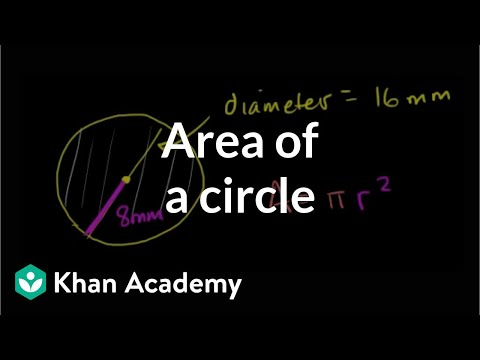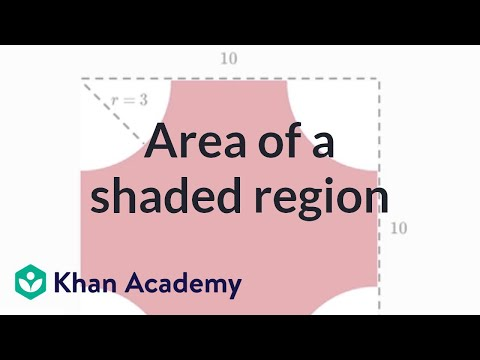Video

# Finding circumference of a circle when given the area (Full video)

Description: Learn how to find the circumference, the distance around a circle, when given the area. Created by Sal Khan. If we know some circle has an area of 36pi can we figure out what the circumference of this circle is? So we know that the area, which is 36pi, is equal to pi r squared. So we could set up an equation pi r squared is equal to 36pi.

### Other videos you might be interested in### Area of a circle (Full video)